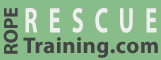# Mass and Force

There are subtle differences between the units that are used to measure mass and force. In an effort to keep RopeRescueTraining.com easy to understand, we often ignore these differences and report both mass and force in pounds and kilograms. However, forces are actually measured in newtons (N), kilograms-force (kgf), or pounds (force) (lbf).

This page explains mass and force, and the units used to measure them, in more detail. This information is mostly theoretical and if it doesn't interest you, feel free to proceed to the next page.

## Mass

"Mass" is a measurement of the amount of material in an object. An object's mass is directly related to the number and type of atoms it contains. In the "International System of Units" (i.e., the "SI" or "metric" system), mass is measured in kilograms. Mass can also be reported in a few other units, including pounds.

The mass of an object remains the same regardless of where the object is located and whether it is moving. An object that has a mass of 100 kg when on the Earth will still have a mass of 100 kg on the Moon—an object's mass can only change by adding or removing material.

## Force

In physics, "force" is the interaction between two objects. If unopposed, force will cause an object's velocity to change. Force can result from air resistance, direct contact, gravity, magnetism, etc.

Newton's second law of motion can be used to define force as the product of an object's mass and its acceleration (i.e., "F=ma"). Force, in SI units, is expressed in newtons.

When calculating the forces in rope systems, the "acceleration" is due to the Earth's gravity which is approximately 9.81 m/s2 (meters per second squared). This means that, ignoring air resistance, a falling object will accelerate 9.81 meters (about 32 feet) per second every second.

So an object that is dropped will be falling at approximately 10 m/s after one second, 20 m/s after two seconds, almost 30 m/s after three seconds, etc. Ignoring air resistance, the rate of acceleration is the same regardless of the object's mass—a feather would fall at the same speed as a rock.

We can use the "F=ma" formula to calculate the static force on a rope by multiplying the mass of the object by 9.81. For example, an object with a mass of 100 kilograms that is hanging on a rope will generate 981 newtons of force (i.e., 100 * 9.81).

This 100 kilogram object is attempting to "accelerate" toward the Earth at 9.81 m/s2, but the gravitational force is being opposed by the rope and anchor. This results in 981 newtons of force on the rope and anchor.

## Weight

In everyday English, when people use the word "weight" they're usually referring to mass—that's how we speak. However, in scientific terms, "weight" is the measurement the force created by an object's mass and gravity.

An object's weight is calculated by multiplying its mass by the acceleration due to gravity.

You can see that both static force and weight are solved by multiplying an object's mass in kilograms by the Earth's gravitational acceleration (9.81 m/s2).

## Measurement Units

As explained above, different units are used when measuring mass than when measuring force. Here is a summary of these measurement units.

• Kilogram (kg). The kilogram measures mass. It is the base unit of mass in the SI (metric) system.
• Kilogram-force (kgf). Kilograms-force is a non-standard unit that measures force. It is equal to the force exerted by the Earth's gravity on a mass of one kilogram.
• Pound. The word "pound" refers to either a unit of mass or force. When the word is used by itself, it is almost always referring to a unit of mass. To reduce confusion, the terms "pound (mass)" and "pound (force)" are used to clarify the measurement unit.
• Pound (mass) (lb or lbm). The pound (mass) measures mass. It is the base unit of mass in the imperial system.
• Pound (force) (lbf). The pound (force) measures force in English Engineering units. It is equal to the force exerted by the Earth's gravity on a mass of one pound.
• Newton (N). The newton measures force. It is the standard unit of force in the SI (metric) system.
• Kilonewton (kN). The kilonewton is a measurement of force. One kilonewton is equal to 1,000 newtons.

## Do words matter?

Does it matter that most rescuers don't make clear distinctions between the units that are used to measure mass and force? If the object is on the Earth, probably not. That's because, as explained above, a kilogram-force is the force exerted by the Earth's gravity on a one-kilogram mass. So, at least on the Earth, the mass and force are equal (even though they are measuring different attributes of the object). If you were on the moon, the mass of this object would still be one kilogram but it would exert only 0.16 kilogram-force.

The basic takeaways are (1) rigging forces are the product of mass and gravitational acceleration, (2) different measurement units are used to express forces and mass, and (3) the difference between force and mass for rescuers on the Earth is mostly theoretical.

vRigger costs less than a rope.